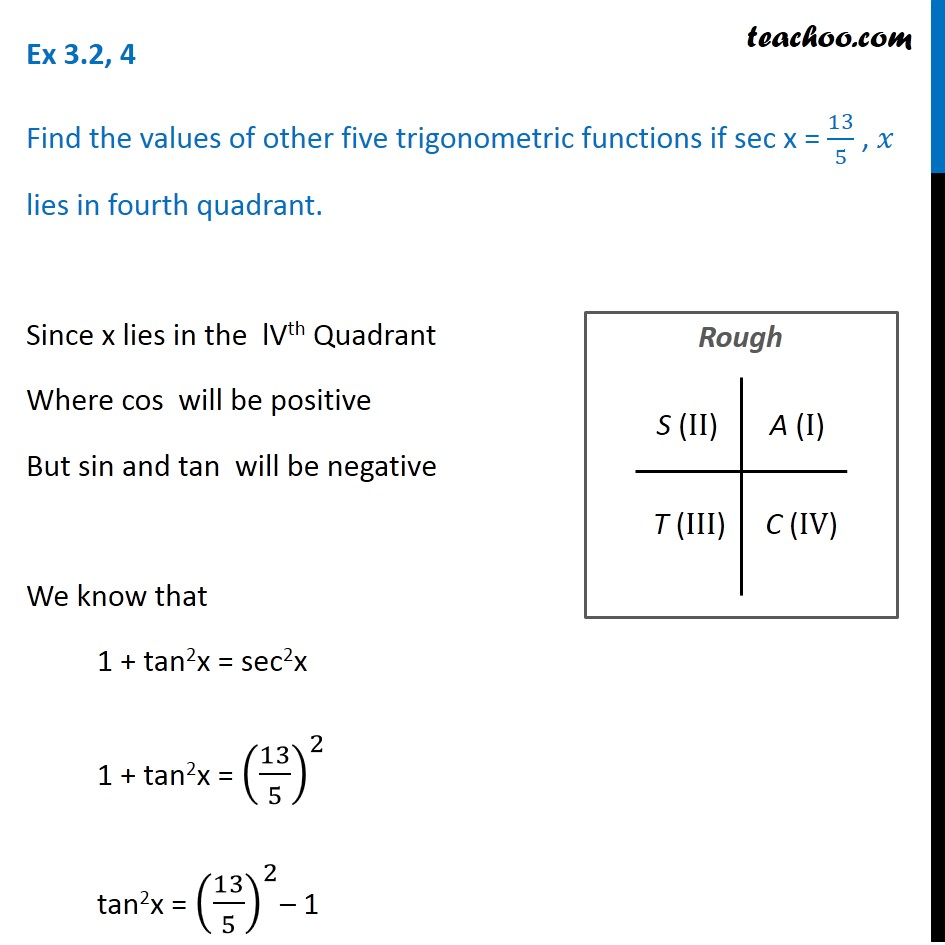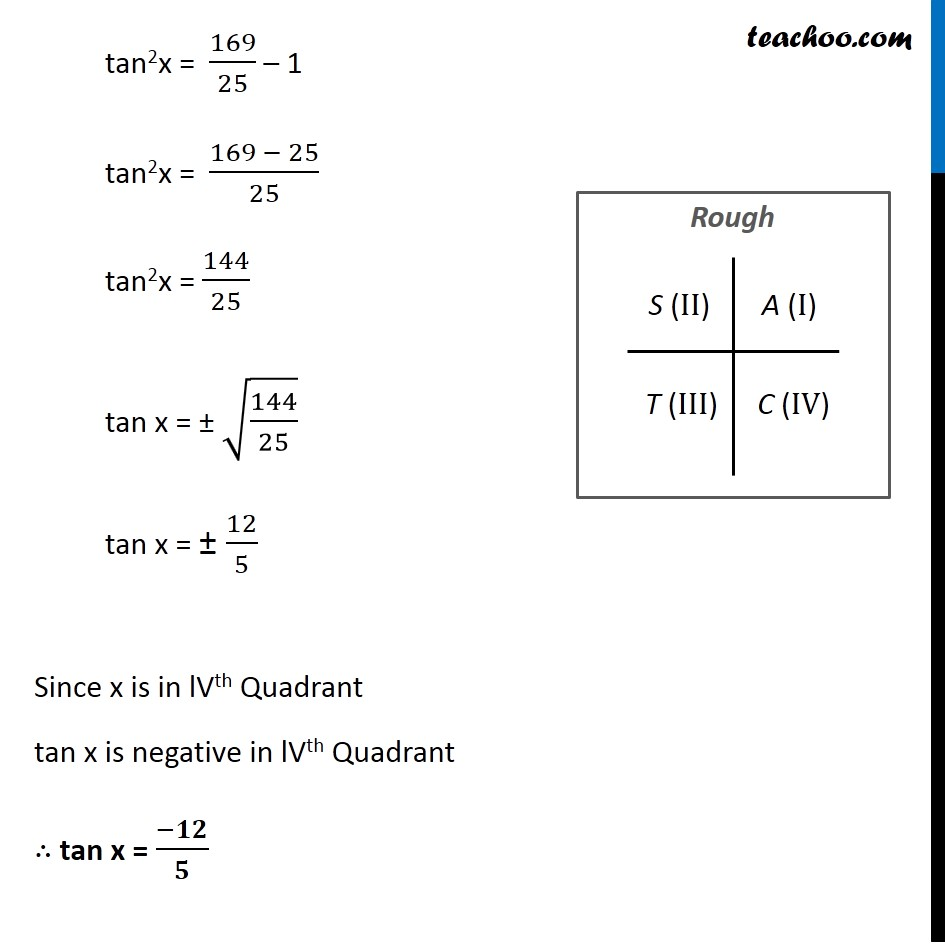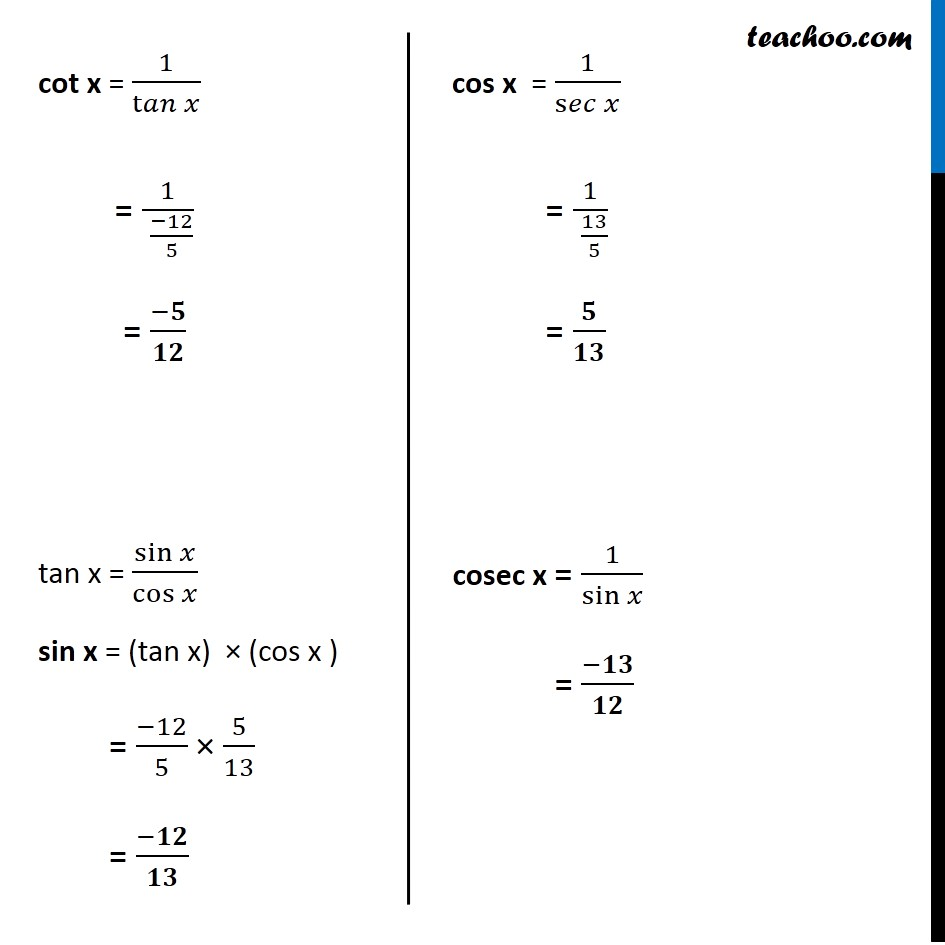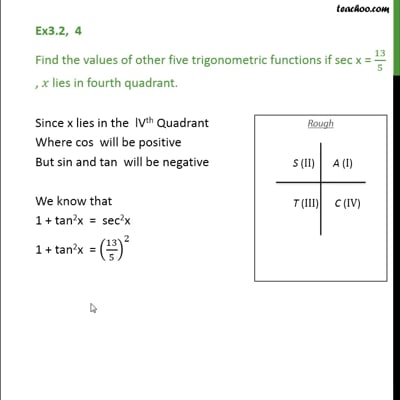Finding Value of trignometric functions, given other functions

Chapter 3 Class 11 Trigonometric Functions
Concept wiseThis video is only available for Teachoo black users

Introducing your new favourite teacher - Teachoo Black, at only ₹83 per month

### Transcript

Ex 3.2, 4 Find the values of other five trigonometric functions if sec x = 13/5 , 𝑥 lies in fourth quadrant. Since x lies in the lVth Quadrant Where cos will be positive But sin and tan will be negative We know that 1 + tan2x = sec2x 1 + tan2x = (13/5)^2 tan2x = (13/5)^2– 1 tan2x = 169/25 – 1 tan2x = (169 − 25)/25 tan2x = 144/25 tan x = ± √(144/25) tan x = ± 12/5 Since x is in lVth Quadrant tan x is negative in lVth Quadrant ∴ tan x = (−𝟏𝟐)/𝟓 cot x = 1/t𝑎𝑛⁡𝑥 = 1/(" " (−12)/5) = (−𝟓)/𝟏𝟐 tan x = sin⁡𝑥/cos⁡𝑥 sin x = (tan x) × (cos x ) = (−12)/5 × 5/13 = (−𝟏𝟐)/𝟏𝟑 cos x = 1/s𝑒𝑐⁡𝑥 = 1/(" " 13/5) = 𝟓/𝟏𝟑 cosec x = 1/sin⁡𝑥 = (−𝟏𝟑)/𝟏𝟐